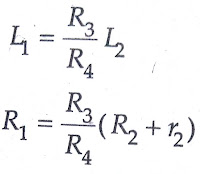# Measurement Of Self Inductance By Maxwell’s Bridge

0
103

## Measurement Of Self Inductance By Maxwell’s Inductance Bridge:

#### Maxwell’s inductance Bridge circuit measures an inductance by comparison with a variable standard self-inductance.The connections and the phasor diagrams for balance conditions are shown in the Maxwell’s Inductance Bridge figure.#### The theory of Maxwell’s Inductance Bridge has been explained already in ac bridges post.### Measurement Of Self Inductance By Maxwell’s Inductance Capacitance Bridge:

#### In Maxwell’s Inductance Capacitance Bridge, an inductance is measured by comparison. with a standard variable capacitance. The connections and the phasor diagram at the balance conditions are given in Maxwell’s Inductance Capacitance Bridge figure below.Let                     L1 = unknown inductance, R1 = effective resistance of inductor L1, R2, R3, R4 = known non-inductive resistances,and       C4 = variable standard capacitor.